# Analysis and Experiment of Heat Transfer Performance of Straight-Channel Grid Regenerator

Analysis and Experiment of Heat Transfer Performance of Straight-Channel Grid Regenerator

Yajuan Wang Jun’an Zhang* Tianle Zhang Zhiwei Lu Hao Dong

School of Mechanical and Electronic Engineering, Xi'an Technological University, Xi'an 710021, Shaanxi, China

Shaanxi Energy Vocational and Technical College, Xianyang 712000, Shaanxi, China

Corresponding Author Email:
zhangjunan@xatu.edu.cn
Page:
781-791
|
DOI:
https://doi.org/10.18280/ijht.400317
11 March 2022
|
Accepted:
4 May 2022
|
Published:
30 June 2022
| Citation

OPEN ACCESS

Abstract:

The design of heat transfer performance of regenerator is the key technology of Stirling machine and is of great significance to ensure the operation efficiency of equipment. In this study the temperature difference between the two ends of regenerator at room temperature is less than 50K. This paper attempts to study the heat transfer performance of the straight channel grid regenerator at room temperature and verify its accuracy. At first, a straight-channel grid regenerator was designed in this study by analyzing the influence of structural parameters on the regenerator in order to reduce the flow resistance loss. The Pore Scale method and Representative Elementary Volume methods were combined to analyze the heat transfer characteristics of the grid regenerator. Compared with the wire mesh regenerator under the same condition, the straight-channel grid regenerator’s flow resistance could reduce by 96.2%. When the length-diameter ratio is 2:1 and the porosity is between 0.4 and 0.5, the heat transfer performance of the grid regenerator is satisfactory when it is applied in Stirling heat pump at room temperature. Then, A segmented variable porosity grid regenerator was designed based on the grid regenerator, and its performance was proved to be better than that of the grid regenerator. Finally, the two regenerators were developed based on additive manufacturing technology, and a single blow experiment platform was built. The experimental results fit well with the simulation results, with the maximum error being less than 1.5%. The experimental results prove the correctness of the model, which lays a foundation for the subsequent analysis of the periodic heat transfer characteristics of the straight-channel grid regenerator.

Keywords:

straight-channel, grid regenerator, flow resistance loss, heat transfer performance

1. Introduction

Free piston Stirling heat pump is a kind of equipment designed on the basis of reverse Stirling cycle. It not only has the advantages of high efficiency of traditional Stirling heat engine, but also cancels the crank connecting rod, which solves the sealing problem, improves the reliability and service life, and has been the focus of domestic and international research. Stirling heat pump can absorb low energy (atmosphere, groundwater and surface water), and the Coefficient of Performance (COP) value is greater than 1. The promotion of energy saving and emission reduction policies has made Stirling heat pump received extensive attention again [1-3].

The temperature difference between hot chamber and cold chamber of Stirling engine is mostly more than 500K [4, 5], for instance, the temperature ratio of the two chambers of 40 HP engine (691K/71K), 4-235 engine (683K/43K), 4-400 engine (633K/41K) and EE-80 engine is 3.0. The temperature difference between the two chambers of Stirling refrigerator in cryogenic temperature range is between 60-300K [6, 7]. The temperature difference between the two ends of Stirling refrigerator in refrigeration temperature range is no less than 100K . The temperature range of Stirling heat pump hot chamber is 290-350K, while the cold chamber temperature is -250-280K at room temperature, and the temperature difference between the two chambers is less than 100K . In terms of temperature range and temperature difference, the Stirling heat pump at room temperature is significantly different from the above types of equipment.

The primary goal of Stirling heat pump is to achieve energy conversion, and the regenerator is the key unit of Stirling heat pump energy conversion. Its efficiency has the most significant impact on the overall efficiency of Stirling heat pump [10-12]. Kato et al.  believe that the efficiency of regenerator depends on the fluctuation of pressure. Yu et al.  point out that the efficiency of the regenerator is related to porosity, thermal permeability depth, number of mesh and materials. Chen’s study  shows that the efficiency of regenerator depends on its material, matrix arrangement, matrix diameter and regenerator filling coefficient. York et al.  studied the effect of wire mesh on neutral pressure drop by numerical method, and found that the pressure loss of a fully aligned regenerator can reduce by 38%-45%. Qiu et al.  conclude that the pressure drops of regenerator accounts for more than 95% of the total pressure drop, and its maximum pressure drop increases almost linearly with the increase of rotational speed. Zhang et al.  estimated three kinds of heat losses in the regenerator at room temperature: conduction loss, incomplete heat loss and viscosity loss caused by pressure drop, and found that the regenerator loss is mainly determined by flow resistance pressure drop loss and axial heat conduction loss, during which flow resistance pressure drop loss accounts for the highest proportion of the total loss.

It can be seen from the above research that the efficiency of regenerator mainly depends on two key performances, namely flow resistance and heat transfer ability. Therefore, aiming to reduce the flow resistance and pressure drop loss of the regenerator in room temperature, two kinds of straight-channel grid regenerator were designed in this study, one of which is called grid regenerator and the other is the segmented variable porosity grid regenerator. The method of combing local Pore Scale and Representative Elementary Volume (REV) was adopted to reveal the main reason influencing the performance of the two regenerators, and the segmented variable porosity grid regenerator can optimize the pressure distribution in the regenerator, which provides a theoretical reference for the design of the regenerator at room temperature.

2. Physical Model of Straight-channel Grid Regenerator

2.1 Grid regenerator

The main factors affecting the structure design of regenerator are length-diameter ratio L/D, wall thickness δ, channel diameter l, specific surface area s, etc. The internal flow resistance loss increases with the regenerator length L when the regenerator diameter D is determined. However, the length L can not be too small, for the reason that the matrix filler is too small to meet the requirements of heat storage. When the length-diameter ratio L/D is determined, the excessively large wall thickness δ or the excessively small channel diameter l will result in a small porosity ϕ and a large flow resistance. When the wall thickness δ is excessively small or the channel diameter l is excessively large, the heat storage will be greatly reduced and the regenerative efficiency will be reduced. The heat transfer area of the regenerator has a positive impact on the heat transfer. The heat transfer area s is affected by wall thickness δ, channel diameter l and porosity ϕ.

Aluminum alloy can be applied as the matrix of regenerator to meet the requirements of heat transfer and storage. In order to reduce the flow resistance loss, a straight-channel grid regenerator with rectangular section is proposed in this article. Compared with the traditional wire mesh regenerator, the regular straight-channel can reduce the flow resistance to the maximum extent. The thickness of the regenerator grid is δ, and the channel length is l. The structure is shown in Figure 1(a)-(b). The uniform wall thickness of δ makes the thermal penetration depth of the matrix material consistent, thus the matrix material can be fully utilized.

## 1.png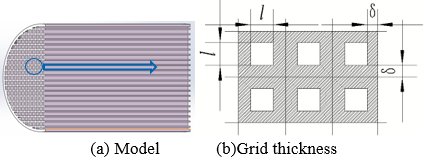Figure 1. 3D Model of the grid regenerator

Thickness δ of the grid regenerator should be less than or equal to the minimum thermal penetration depth δs, which means that δδs, the minimum thermal penetration depth δs is written as Eq. (1):

$\delta_{s}=\sqrt{\left(\lambda_{w} / \pi f \rho c_{p}\right)}$  (1)

The calculated minimum thermal penetration depth δs is 0.8mm, which means the thickness of the grid can’t exceed 0.4mm.

The minimum thermal penetration depth of working medium δv is expressed as Eq. (2):

$\delta_{v}=\sqrt{\left(\lambda_{w} / \pi f \rho\right)}$  (2)

where, δv  is 1.47 mm obtained through calculation, thus the channel diameter l is taken as 1.5mm.

Considering the uniformity of heat transfer, the flow channel was designed as square of 1.5mm× 1.5mm length. Requirements of regenerator resistance and heat storage were considered comprehensively, the length-diameter was set as 2:1, and the specific design parameters are shown in Table 1.

Table 1. Parameters of the grid regenerator

 Parameter value Diameter D(mm) 40 Length L(mm) 80 Minimum heat transfer depth δs(mm) 0.4 Side length of a regular quadrilateral l(mm) 1.5 Diameter-Length ratio L/D 2:1 Porosity ϕ 0.446 Grid thickness δ(mm) 0.4 Material Aluminum alloy

2.2 Segmented variable porosity grid regenerator

According to the heat transfer characteristics of the hot end and the cold end, the heat transfer efficiency varies with the porosity. Based on the grid regenerator, a segmented variable porosity grid regenerator is further proposed, as shown in Figure 2. Figure 3(a)~(d) illustrates the sectional diagram of the regenerator, which contains three grids, two grids, one grid and zero grid, respectively.

## 2.png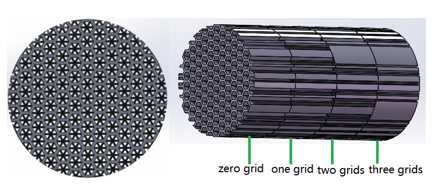Figure 2. 3D Model of segmented variable porosity grid regenerator

## 3.pngFigure 3. Sectional diagram of the regenerator

Based on the structural parameters of the grid regenerator (Table 1), a segmented variable porosity grid regenerator with the same length-diameter ratio and porosity is proposed, with its structural parameters shown in Table 2. From Figure 4, it can be found that the porosity is axially distributed.

Table 2. Structure parameter

 Parameter value Diameter D(mm) 40 Total length L (mm) 80 Porosity of three grids 0.261 Porosity of one grid 0.51 Average porosity 0.446 Length of each part(mm) 20 Grid thickness δ (mm) 0.4 Side length of a regular hexagon l (mm) 1.65 Porosity of two grids 0.376 Porosity of zero grid 0.647 Minimum heat transfer depth δs (mm) 0.4 Material Aluminum alloy

## 4.pngFigure 4. Axially distributed of Porosity

3. Governing Equations and Flow of Regenerator Heat Transfer Performance analysis

3.1 Governing equations for local Pore Scale analysis

Regenerator is porous medium. Generally, porous medium numerical approach is adopted for simulation analysis . In this study, two methods were adopted for the simulation of flow and heat transfer, namely Pore Scale method and REV. In the analysis and calculation of the Pore Scale method, the local porous medium was selected as the research object first, and then a three-dimensional model of the solid skeleton was established to study the flow and heat transfer law between the skeleton and the surrounding fluid. The REV method is a continuum medium method, which overcomes the deficiency of Pore Scale method in engineering application. Due to the introduction of the concepts of porosity and REV method, the porous medium was assumed as continuum to study the macroscopic flow and heat transfer phenomenon. Semi-empirical correlations such as pressure drop correlations and heat transfer correlations were involved in the REV method, and these empirical correlations must be obtained by means of Pore Scale method.

Based on the established local model, the standard k-ε turbulence model and the second order upwind scheme discrete control equation were adopted to calculate. The discrete equation of the second order upwind scheme has an intercept of second order accuracy, which can not only guarantee the accuracy of calculation, but also reduce the diffusion error obviously. In the standard k-ε model, k and ε are two basic unknowns, and the corresponding transport equations are shown as Eqns. (3)-(4):

$\frac{\partial(\rho k)}{\partial t}+\frac{\partial\left(\rho k u_{i}\right)}{\partial x_{i}}$$=\frac{\partial}{\partial x_{j}}\left[\left(\mu+\frac{\mu_{i}}{\sigma_{k}}\right) \frac{\partial k}{\partial x_{j}}\right]+G_{k}+G_{b}-\rho \varepsilon-Y_{M}+S_{k}$   (3)

$\frac{\partial(\rho \varepsilon)}{\partial t}+\frac{\partial\left(\rho \varepsilon u_{i}\right)}{\partial x_{i}}=\frac{\partial}{\partial x_{j}}\left[\left(\mu+\frac{\mu_{i}}{\sigma_{\varepsilon}}\right) \frac{\partial \varepsilon}{\partial x_{j}}\right]$

$+G_{1_{\varepsilon}} \frac{\varepsilon}{k}\left(G_{k}+G_{3 \varepsilon} G_{h}\right)-G_{2 c} \rho \frac{\varepsilon^{2}}{k}+S_{g}$   (4)

The working medium is incompressible, whose physical property parameters (density, viscosity, specific heat capacity and thermal conductivity) remain unchanged at any temperature. The energy equation on one-dimensional flow is defined as Eq. (5):

$\frac{\partial}{\partial t}(\rho E)+\frac{\partial}{\partial x}(u(\rho E+P)+q)-Q=0$  (5)

According to the energy Eq. (5), it can be deduced that under the condition of convection heat transfer between the matrix and the working medium, the heat transfer Q can be calculated with Eq. (6):

$Q=h A\left(T_{r}-T_{g}\right)=m c_{p} \Delta T_{g}$  (6)

The convective heat transfer coefficient h can be obtained from Eq. (6), as shown in the following Eq. (7):

$h=m c_{p} \Delta T_{g} / A \Delta T_{m}$  (7)

where: $\Delta T_{\mathrm{m}}$ can be calculated with the arithmetic average method, and the flow resistance coefficient f can be calculated with Eq. (8):

$f=\frac{d}{L} \cdot \frac{\Delta p}{0.5 \rho u_{\max }^{2}}$  (8)

The relationship between unidirectional flow resistance coefficient f and Reynolds number Re is defined as Eq. (9):

$f=a / \operatorname{Re}+b / \operatorname{Re}^{c}$  （9)

where, parameters a, b, c can be determined by fitting curves.

According to the research report of Liu et al. , the relationship between regenerator speed and pressure drop can be calculated by the Darcy Forchheimer’s Eq. (10):

$\frac{d p}{d x}=\frac{\mu}{K} v+R_{f} \rho v^{2}$  (10)

3.2 Governing equations of REV analysis

The fluid-solid phase in porous medium was assumed to be continuous phase, the heat transfer process was numerically. simulated based on the porous medium model, and the inertia resistance coefficient and viscous resistance coefficient were obtained. The velocity field and temperature field of gas in porous medium were analyzed through the introduction of porosity and resistance coefficient. The momentum equation is written as Eq. (11):

$\frac{\partial \left( \rho \overset{\to }{\mathop{u}}\, \right)}{\partial t}+\nabla \bullet \left( \rho \overset{\to }{\mathop{u}}\,\overset{\to }{\mathop{u}}\, \right)=\text{-}\nabla P+\nabla \bullet \left( \overline{\overline{\tau }} \right)+\rho \overset{\to }{\mathop{g}}\,+{{S}_{i}}=0$  (11)

The expression of Siis Eq. (12):

$S_{i}=\frac{u}{c_{f}} u+\frac{\mathrm{c}_{\mathrm{j}}}{2} \rho u^{2}$  (12)

The source term acting on the flow body produces a certain pressure gradient, and the pressure drop inside the regenerator can be obtained with Eq. (13):

$\nabla p=S_{\mathrm{i}} \Delta n$  (13)

where, Δn is the length of porous medium.

3.3 Analysis flow of heat transfer performance of regenerator

The logic flowchart is shown in Figure 5. Firstly, the influence factors of Stirling regenerator at room temperature were analyzed, and the main factors affecting the performance of Stirling regenerator were summarized as length-diameter ratio and porosity, and the structure of Stirling regenerator was designed. Secondly, a 3D model of local regenerator based on Pore Scale was established. By analyzing the relationship between pressure and velocity and substituting it into the representational volume element scale, the porous medium model was established, and the pressure distribution law of the regenerator was obtained. The flow resistance and pressure drop of the regenerator was lower than that of the regenerator with the same porosity. Finally, the regenerator of length to diameter ratio and porosity structure parameter optimization, a new type of regenerator with different grid and porosity was proposed Based on the 3 D model of local regenerator and characterization of the Pore Scale element of porous media model to analyze the segmented grid regenerator and its flow resistance pressure drop is much better. The rationality and effectiveness of grid and segmented grid regenerator are verified.

## a.png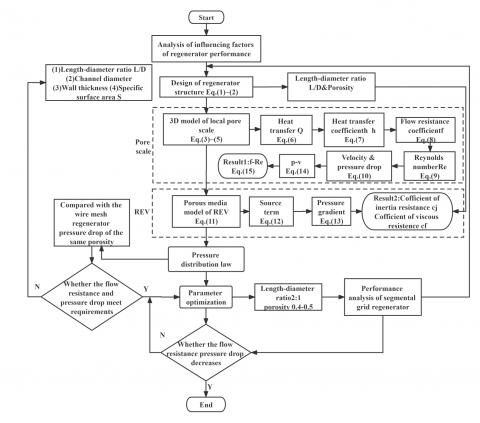Figure 5. The logic flowchart of this work

4. Numerical Analysis of Heat Transfer Performance of the Regenerators

4.1 Numerical analysis of the Pore Scale of grid regenerator

4.1.1 Local 3D geometric model

Four holes of the regenerator were retrieved for calculation to reduce the back flow effect of the inlet and the outlet, which were set 5mm away from the regenerator (verified by CFD simulation), making a certain distance from the matrix. Local 3D model is shown in Figure 6, which presents that the red interface is the inlet of the working fluid, while the blue interface is the outlet boundary, and the gray is the area surrounded by the gas domain of the regenerator matrix.

## 6.jpgFigure 6. Local three-dimensional model

The mesh was divided by tetrahedral non-structure, with its surrounding mesh being encrypted. The total number of mesh is more than 3 million with good quality. ICEM software is used to conduct tetrahedral mesh division on the model (Figure. 6). The model is a regular cuboid channel, structure of which is very simple. Therefore, the uniformity of grid division is very good, and the preliminary prediction result of simulation has fine quality, and it is not sensitive to the changes of grid numbers and grid scale. The boundary conditions are shown in the following Table 3:

Table 3. Boundary conditions

 Inlet boundary Constant velocity inlet, temperature stabilization 285K Outlet boundary Open borders 325K Boundary wall Symmetrical wall Internal matrix wall Fluid-solid coupling wall, no slip

4.1.2 Numerical calculating results

With air being the working medium, aluminum alloy being the matrix material, and the inflation pressure being 1.5MPa, the gas flow and heat transfer of the regenerator in the process of hot single blowing were simulated. In the process of hot blowing, the speed inlet was set at 5m/s and the temperature remained constant at 325K. The outlet is an open boundary with the ambient temperature remaining constant at 285K. The three-dimensional temperature distribution of hot blowing can be referred in Figure 7.

## 7.pngFigure 7. Three-dimensional temperature distribution during hot blowing

## 8.pngFigure 8. Single-channel temperature distribution cloud diagram for 5s hot blowing

Heat transfer of one channel under 5s hot blowing is illustrated in Figure 8.

Afterwards, cold blowing simulation was carried out with the initial temperature of the matrix being 321K at the end of hot single blowing, and the working medium temperature is 285K during cold blowing. Figure 9 shows the 3D temperature distribution during cold blowing.

## 9.pngFigure 9. 3D temperature distribution during cold blowing

Heat transfer of a channel under 5s cold blowing is shown in Figure 10.

## 10.png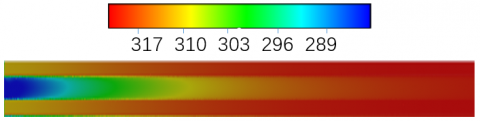Figure 10. Single-channel temperature distribution cloud diagram for 5s cold blowing

Figure 7 and 8 show that when the high-temperature working fluid flows through the regenerator, the internal temperature drops in a stepped manner. The temperature of the regenerator matrix increases with time and finally reaches the critical value, without heat exchange between the working fluid and the matrix anymore. From Figure 9 and 10, it can be noted that after the ambient temperature working fluid passing through the regenerator, the working fluid absorbs the heat stored in the matrix. With the rise of temperature, the temperature of the matrix gradually decreases, and the heat stored in the matrix is gradually absorbed. The regenerator gradually cools to the ambient temperature with the increase of time.

As shown in Figure 11, the model is used to solve the pressure gradient of working materials at different inlet speeds. The fitting equation of velocity and pressure drop is obtained from Figure 11, as presented in Eq. (14):

ΔP=229.7v+4.9061v2  (14)

Heat transfer inside the regenerator changes with temperature, speed and pressure. Ergun  obtained the correlation formula of friction factors: $f=\frac{1-\varepsilon }{{{\varepsilon }^{2}}}(\frac{150}{\operatorname{Re}}+1.75)$ (Re<3000), while Macdonald  revised this formula into $f=\frac{1-\varepsilon }{{{\varepsilon }^{2}}}(\frac{150}{\operatorname{Re}}+b)$, in which b is related to the surface smoothness. Tanaka  calculated the change rule of regenerator flow resistance coefficient f with Re number through experiments under atmospheric pressure: $f=175/\operatorname{Re}+1.6$ (0.1-1Mpa). Comparative analysis was conducted on the simulated grid regenerator under atmospheric pressure with Tanaka's experiment, and the results are shown in Figure 12.

## 11.pngFigure 11. p-v curve

## 12.pngFigure 12. f-Re relation unidirectional flow

Figure 12 illustrates the fitting relation (Eq. (15)) of unidirectional flow resistance coefficient f and Reynolds number Re. The comparison result shows that the two has a high degree of coincidence. In Figure 12, compared with other points, point 2 deviates the largest distance from Tanaka curve. Therefore, f value of point 2 is compared with Tanaka value of the same Re for calculation, and the maximum error difference is less than 5.23%.

$f=2013/\operatorname{Re}\text{-}1.469$  (15)

4.2 Numerical analysis and optimization of REV of grid regenerator

4.2.1 Numerical analysis

According to the formula of velocity and pressure drop (Eq. (11)), the formula of source term (Eq. (12)) and the formula of internal pressure drop of the regenerator (Eq. (13)), combined with the numerical simulation results of the local three-dimensional model (Eqns. (14-15)), the inertia resistance coefficient of the regenerator cj and the viscous resistance coefficient cf can be calculated, with cj being 100.12 and cf being 1.6108, respectively. A 2D regenerator porous medium model was established, and the calculated resistance coefficient cj, cf and porosity ϕ were brought into the porous medium model. In unidirectional flow, the inlet boundary was defined as the velocity boundary, the outlet as the open boundary, and the wall surface as the non-slip boundary. The pressure distribution rule of the grid regenerator is shown in Figure 13(a). Figure 13(b) presents the pressure of wire mesh regenerator with the same porosity, and the inertial resistance coefficient and viscous resistance are 253 and 5.79×109, respectively .

## 13a.png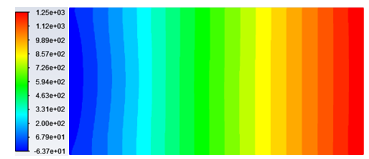(a) Pressure distribution of gird regenerator

## 13b.png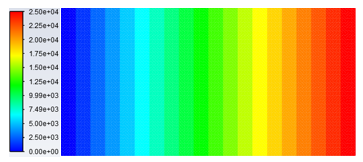(b)Pressure distribution of wire mesh regenerator

Figure 13. Pressure

The result of comparison between the grid regenerator and the wire mesh regenerator with the same porosity shows that flow resistance of the grid regenerator is 96.2% lower than that of the mesh regenerator, as shown in Figure 14, indicating that the straight-channel of the grid regenerator can effectively reduce the flow resistance loss at room temperature.

## 14.pngFigure 14. Flow resistance loss comparison

4.2.2 Optimization of main parameters

The main parameters affecting the regenerator structure include length-diameter ratio, wall thickness, etc., which can be optimized by length-diameter ratio and porosity. Figures 15 and 16 present the rule of length-diameter ratio and porosity on flow resistance pressure drop loss of grid regenerator through simulation analysis. The pressure drop of regenerator changes linearly, as shown in Figure 15(a). When the length-diameter ratio is 3:2, the pressure drop is the smallest, followed by the ratio of 2:1, and when the pressure drop of the regenerator is 3:1, the pressure drop is the largest, as shown in Figure 15(b). Therefore, with the consideration of the change of pressure drop and heat storage performance, the length-diameter ratio of 2:1 is optimum.

The porosity of the wire mesh regenerator is generally between 0.6-0.8. For the grid regenerator, the porosity is of research value between 0.4-0.7 because of its straight-channel structure. When the porosity is less than 0.4, the regenerator flow resistance is too large, and when the porosity is greater than 0.7, the regenerator flow resistance is small but the heat storage capacity is poor. What’s more, the pressure drop inside the gird regenerator decreases linearly. The maximum pressure occurs when the porosity is 0.4, then 0.5, 0.6 and 0.7 successively. The pressure of a porosity between 0.4 to 0.5 can minimize the flow resistance of the grid regenerator compared to other porosities.

## 15a.png(a) Internal pressure drop

## 15b.png(b) Change of length-diameter ratio

Figure 15. Influence of length-diameter ratio on pressure drop

## 16.pngFigure 16. Pressure drop of different porosity

4.3 Numerical analysis of segmented grid regenerator

The segmented variable porosity grid regenerator was proposed on the basis of the grid regenerator, aiming to improve the distribution of pressure drop in the grid regenerator. The internal heat transfer characteristic of the variable porosity regenerator can be solved by Pore Scale method, and the inertial resistance coefficient cj and viscous resistance coefficient cf of the segmented variable porosity regenerator can be obtained by the REV method. The results are shown in Table 4.

Table 4. Porosity and heat transfer coefficient

 Segment Length l (mm) Porosity  ϕ Inertia resistance coefficient cj Viscous resistance coefficient cf Zero grid 20 0.647 79.024 4.02×105 One grid 20 0.510 202.9388 2.63×106 Two grids 20 0.376 403.5918 1.29×107 Three grids 20 0.261 987.7551 1.8×107

The internal pressure drop varies with the connection mode of the segmented variable porosity grid regenerator. Figure 17 illustrates the pressure drop change of the regenerator when the hot end is connected with three grids and zero grid respectively. Regenerator porosity of the regenerator has a great influence on the pressure drop. For example, when the hot end is connected to the zero grid which has the large porosity, the flow resistance of the regenerator becomes small, thus the pressure drop is small. As the porosity decreases, the flow resistance loss increases and the pressure drop of the regenerator also increases. The pressure drop of the connection between the hot end and the three grids is always less than that between the hot end and the zero grid.

## 17.png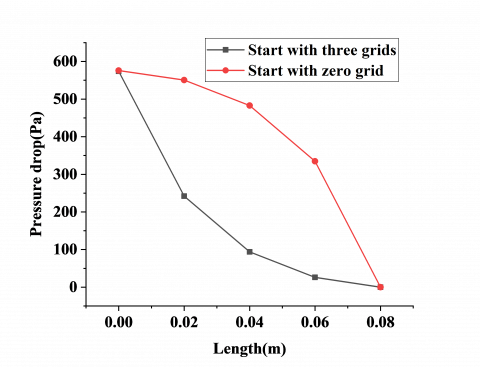Figure 17. Pressure drop curve of the segmented variable porosity grid regenerator with different connection

5. Experimental Analysis

5.1 Experimental principle and scheme

The principle of the experiment is shown in Figure 18(a). The compressed air was fed into the copper tube, which was heated by the copper tube and then sent into the regenerator. Two thermocouples were arranged at the inlet and outlet of the regenerator to collect temperature changes at both ends, the collected signal was sent to the computer by data acquisition card. Since it was difficult to complete data measurement in the closed system, the open experiment system was adopted in this study. The schematic diagram of the experimental device is shown in Figure 18(b): the two regenerators designed for the device are shown in Figure 19(a)-(b), the experimental device is connected with high pressure air source, regenerator, flow meter, heating copper pipe, intermediate pipe and Labview temperature acquisition system, its main measuring instruments are shown in Table 5. Limited by experimental conditions, it is difficult to heat the air and make its temperature change stably. By spiraling the copper tube, the air is heated by water bath heating method. In order to demonstrate the integrity of the experimental equipment, copper tubes are placed on the table from the heated tank as shown in Figure 18(b). The experiment was carried out at room temperature, and the flow rate could be adjusted by the throttle valve and the gas mass flow meter. The mass flow rate could not exceed 150slm. Because the temperature detected by the thermocouple is not the direct temperature change, but the potential change of the thermocouple, 1K corresponds to 41mV, so the accuracy of the collected data can reach 10-5.

Table 5. Main measuring instruments

 Experimental apparatus Type Range precision Digital gas mass flow meter GMFC-CXB-4- R4-D-A1-F2 30-150slm 1% K-type Thermocouple customize 73-1743K A level DAQ Card PCI-8192 Precision thermocouple amplifier AD8495 10-5

## 18a.png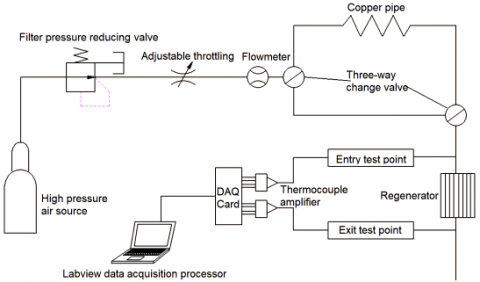(a) Experiment scheme

## 18b.png(b) Pipe connection layout

Figure 18. Experimental facility

## 19.png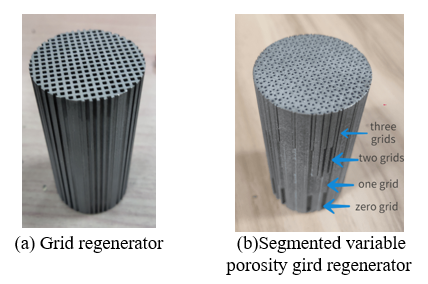Figure 19. Regenerator physical object

Those small pores made it difficult to adopt traditional processing methods. The actual processing objects were produced on the basis of additive manufacturing technology, which are shown in Figure 19(a) and Figure 19(b).

5.2 Impact of systematic error and random error

The influence of systematic error on the experiment is to make the experimental value detected high or low, there may be two reasons, the first one is thermocouple, which can be reduced by calibrating the thermocouples before experiment. The second is the regenerator, its cylindrical surface is in direct contact with room temperature without surface insulation, which leads to systematic errors in the experimental process. In all, the systematic errors would influence the final experimental value.

Due to the influence of environmental temperature fluctuation (the copper pipe is electrically heated by the tank) and other factors, the random error is caused. The frequency of signal collection is 20KHz, and the average value of each collected signal is calculated. After several measurements, it is found that they can cancel each other, so the influence caused by random error can be effectively reduced.

5.3 Experimental results

In order to verify the accuracy of the above simulations, the inlet parameters measured through the experiment were taken as the inlet boundary conditions of the numerical model, and the corresponding outlet temperature was obtained after the numerical simulation and compared with the experimental test value. With the same flow rate, the unidirectional flow experiment of the two regenerators were conducted to obtain the experimental value of outlet temperature. The analysis results of the comparison between the experimental value and the simulation value are shown in Figures 20-21.

## 20a.png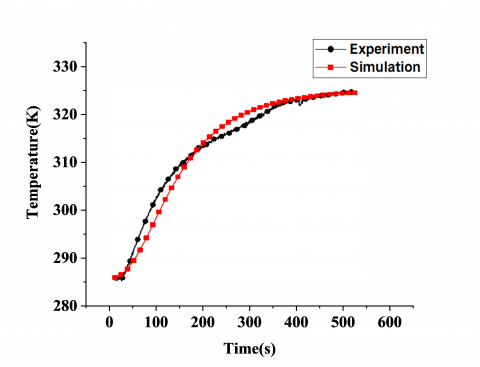(a) Hot blowing

## 20b.png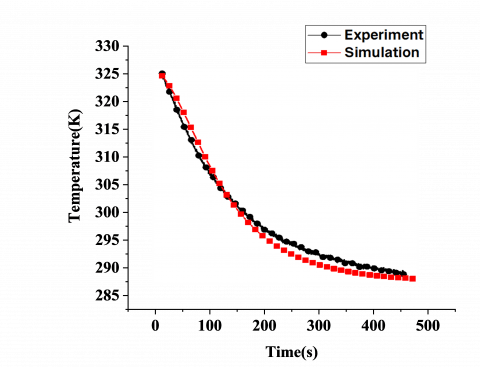(b) Cold blowing

Figure 20. Simulation and experimental curves of outlet temperature of the gird regenerator

## 21a.png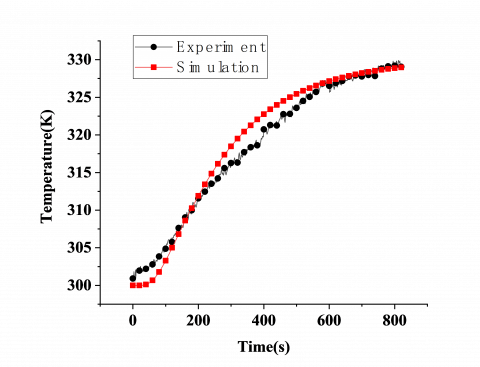(a) Hot blowing

## 21b.png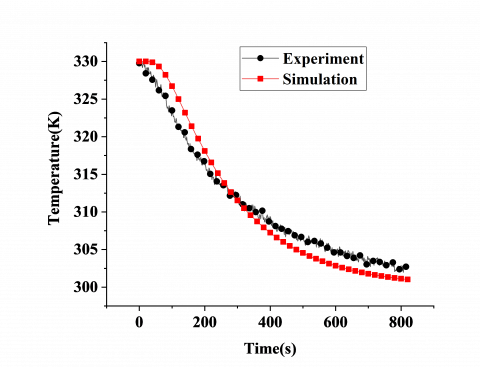(b) Cold blowing

Figure 21. Simulation and experimental curves of outlet temperature of the segmented variable porosity grid regenerator

5.4 Analysis of experimental results

With the same material and porosity, the pressure drop of the segmented grid regenerator is 470Pa smaller than that of the grid regenerator (Figure 14 and Figure 17). With the same flow rate, the heat transfer time of the segmented grid regenerator is 820s, while the grid regenerator is 600s, which means that the grid regenerator can reach the heat balance faster, but the heat transfer is small, whereas the segmented variable grid regenerator warms up slowly, but the heat transfer is large (Figure 20 and Figure 21). The result indicates that the heat transfer performance of the segmented variable porosity gird regenerator with the same material and porosity is better than that of the grid regenerator.

As can be seen from Figure 20(a)-(b) to Figure 21(a)-(b), the experimental results of the two regenerators are in good agreement with the simulation results, although there is a slight difference because the regenerator is not insulated and there is heat exchange with the outside world. The so-called system deviation makes the experimental curve and the simulation curve do not coincide exactly, the two curves have the following rules: at the early stage of hot blowing, the simulated value of outlet temperature of regenerator is higher than the experimental value, and then the experimental value is higher than the simulated value with the increase of hot blowing time, finally, the experimental value is consistent with the simulation value. At the early stage of cold blowing, the simulation value of the outlet temperature is higher than the experimental value, and as the further cold blowing continues, the experimental value of the regenerator is higher than the simulation value, the experimental value is consistent with the simulation value at last.

At the early stage of hot blowing, the temperature of the regenerator is low and there is no heat exchange, so the experiment value is higher than the simulation value. With the increasing temperature of the regenerator, there is heat exchange between the regenerator and the outside world, and part of the absorbed heat is released to the outside world, so the experiment value is lower than the simulation value. As the heating continues, the heat absorbed by the regenerator gradually slows down, and there is no heat exchange between the regenerator and the outside world. Finally, the experimental and simulated values of outlet temperature tend to be stable and consistent, and the cold blowing curves change similarly after endothermic process. The cold blowing curve after endothermic process is also related to the heat exchange with the outside world.

The experimental results are in good agreement with the simulation results under hot and cold blowing, with the error controlled within 1.5%, which verifies the correctness of the theoretical simulation analysis and provides a research basis for the optimization of the regenerator at room temperature.

6. Conclusion

In this study, a straight-channel grid regenerator for Stirling heat pump at room temperature was designed, and the real object was produced based on additive manufacturing technology. The heat transfer performance of the straight-channel grid regenerator was revealed through the research.

On the basis of the research results and the relevant analysis, the following conclusions were arrived at:

(1) In order to reduce the flow resistance loss, the influence of parameters including length-diameter ratio, wall thickness, diameter, etc. on the regenerator structure was analyzed. With full consideration of the requirements of thermal penetration depth of matrix and gas, a regenerator with straight-channel structure was designed. Through the simulation analysis, it was found that the flow resistance loss reduces by 96.2% compared with the mesh regenerator with the same porosity.

(2) The correlation formula of friction coefficient of grid regenerator under unidirectional flow was established, and the error between the experimental values under the same condition is not more than 5.23%, which has verified the universality of grid regenerator in Stirling heat pump.

(3) When the length-diameter ratio of the regenerator is 2:1 and the porosity is between 0.4-0.5, the grid regenerator has the best performance, and the pressure drop inside the grid regenerator is linear. The segmented variable porosity grid regenerator was proposed on the basis of grid regenerator. The pressure distribution of the segmented variable porosity grid regenerator has been effectively improved by the segmented variable structure, and the pressure drop of each segment is linear. This structure can greatly increase the heat transfer performance compared with the grid regenerator.

(4) The maximum error between the simulation and experimental values of the regenerator under unidirectional flow is less than 1.5%, which has verified the correctness of the theoretical analysis method, and has laid a foundation for the subsequent performance research on the regenerator oscillating flow.

Acknowledgements

This work is supported by the National Natural Science Foundation of China (No.51705390), Natural Science Basic Research Program of Shaanxi Province (General Program) (No.2020JM-720), and "Coal Mine Intelligent Machinery Equipment Research and Innovation Team (2021KYTD04)".

Nomenclature
 l the channel length, mm λw fluid thermal conductivity, W/m K cp specific heat capacity at constant pressure, kJ/(kg K) f frequency, Hz Gk the generation term of turbulent kinetic energy k caused by the average velocity gradient Gb the generation term of turbulent kinetic energy k caused by buoyancy YM pulsating expansion in compressible turbulence σk Pr numbers corresponding to turbulent kinetic energy k σε Pr numbers corresponding to dissipation rate ε Sk and Sg user-defined source entries E total energy, J u the velocity component in the x direction, m/s q heat flow, W/m2 Q heat transfer, W P pressure, Pa $\Delta T_{\mathrm{g}}$ temperature difference between inlet and outlet of gas, K $\Delta T_{\mathrm{m}}$ the temperature difference between gas and solid, K h convective heat transfer coefficient, W/(m2 K) Re Reynolds number k permeability Rf inertia coefficient. $\overline{\overline{\tau }}$ stress tensor $\rho \overset{\to }{\mathop{g}}\,$ gravity Si source term Δn length of porous medium, mm cf viscous resistance coefficient of porous media cj coefficient of inertia resistance of porous media Greek symbols δ thickness of the grid regenerator, mm δs the minimum thermal penetration depth, mm δv The minimum thermal penetration depth of working medium ρ fluid density, kg/m3
References

 Hachem, H., Gueith, R., Aloui, F., Dincer, I., Nasrallah, S.B. (2015). Energetic and exergetic performance evaluations of an experimental beta type Stirling machine. In: Dincer I., Colpan C., Kizilkan O., Ezan M. (eds) Progress in Clean Energy, Volume 2. Springer, Cham. https://doi.org/10.1007/978-3-319-17031-2_51

 Li, K., Yu, G.Y., Zhang. Y.B., Liao,X., Dai, W., Luo., E.C. (2015). Theoretical and experimental research on free piston Stirling heat pump. Journal of Engineering Thermophysic, 36(01): 41-45.

 Wang, Y.J., Zhang, J.A., Liu, B., Dong, H., Liu, X.Y. (2021). Progress in application of reverse Stirling cycle technology in air conditioning system of pure electric vehicle. Journal of Xi’an Technology University, 41(6): 609-620. https://doi.org/10.16185/j.jxatu.edu.cn.2021.06.001

 Kim, S., Huth. J., Wood, J. (2005). Performance characterization of sunpower free-piston Stirling engines. 3rd International Energy Conversion Engineering Conference, San Francisco, California, AIAA 2005-5540. https://doi.org/10.2514/6.2005-5540

 Wood, J.G., Lane, N. (2003). Advanced 35 W free‐piston Stirling engine for space power applications. AIP Conference Proceedings. American Institute of Physics, 654(1): 662-667. https://doi.org/10.1063/1.1541353

 Tlili, I. (2012). Finite time thermodynamic evaluation of endoreversible Stirling heat engine at maximum power conditions. Renewable & Sustainable Energy Reviews, 16(4): 2234-2241. https://doi.org/10.1016/j.rser.2012.01.022

 Tlili, I., Timoumi, Y., Nasrallah, S.B. (2008). Analysis and design consideration of mean temperature differential Stirling engine for solar application. Renewable Energy, 33(8): 1911-1921. https://doi.org/10.1016/j.renene.2007.09.024

 Cui, Y.H., Qiao, J.X., Wang, X.T., Song, B., Yang Z.H., Dai, W., Li, H.B. (2021). Stirling cooler operating in room temperature. CIESC Journal, 72(S1): 390-397. https://doi.org/10.11949/0438-1157.20201580

 Chen, X., Ling, F., Liu, X., Peng, W.T., Huo, Q.Z., Yang, W.L., Yang, J.Q. (2019). Optimization design of regenerator of free piston Stirling cryocooler in air-conditioning temperature zone. Vacuum & Cryogenics, 25(04): 231-236. https://doi.org/10.3969/j.issn.1006-7086.2019.04.003

 Ataer, Ö.E., Karabulut, H. (2005). Thermodynamic analysis of the V-type Stirling-cycle refrigerator. International Journal of Refrigeration, 28(2): 183-189. https://doi.org/10.1016/j.ijrefrig.2004.06.004

 Rogdakis, E., Antonakos, G., Koronaki, I.P. (2016). Influence of a regeneror on engine performance. Journal of Energy Engineering, 142(2): E4016002. https://doi.org/10.1061/(ASCE)EY.1943-7897.0000338

 Canazas, J. (2021). Field study on the air-side heat transfer performance of copper finned-flat tubes for heavy-duty truck radiators. International Journal of Heat and Technology, 39(5): 1451-1459. https://doi.org/10.18280/ijht.390506

 Kato, Y., Baba, K. (2014). Empirical estimation of regenerator efficiency for a low temperature differential Stirling engine. Renewable Energy, 62: 285-292. https://doi.org/10.1016/j.renene.2013.07.023

 Yu, Y.J., Cheng, C.H. (2010). Dynamic simulation of a beta-type Stirling engine with cam-drive mechanism. ASME International Mechanical Engineering Congress and Exposition, 44298: 655-664. https://doi.org/10.1115/IMECE2010-40635

 Chen, W.L., Wong, K.L., Chen, H.E. (2014). An experimental study on the performance of the moving regenerator for a γ-type twin power piston Stirling engine. Energy Conversion and Management, 77: 118-128. https://doi.org/10.1016/j.enconman.2013.09.030

 York, B.T., Macdonald, B.D. (2021). Influence of misalignment and spacing on the pressure drop through wire mesh Stirling engine regenerators. Energy Conversion and Management, 245(2): 114588. https://doi.org/10.1016/j.enconman.2021.114588

 Qiu, H., Wang, K., Yu, P., Ni, M.J.,Xiao, G. (2021). A third-order numerical model and transient characterization of a β-type Stirling engine. Energy, 222: 119973. https://doi.org/10.1016/j.energy.2021.119973

 Zhang, T.L., Zhang, J.A., Wang, Y.J., Liu, B. (2021). Design and analysis of new type regenerator for Stirling engine. CRYOGENICS, 2: 18-24. https://doi.org/10.3969/j.issn.1000-6516.2021.02.004

 Faruoli, M., Viggiano, A., Magi, V. (2019). A porous media numerical approach for the simulation of Stirling engine regenerators. TECNICA ITALIANA-Italian Journal of Engineering Science, 63(2-4): 291-296. https://doi.org/10.18280/ti-ijes.632-425

 Liu, Y.P., Xu, G.Q., Luo, X., Li, H.W., Ma, J.D. (2015). An experimental investigation on fluid flow and heat transfer characteristics of sintered woven wire mesh structures. Applied Thermal Engineering, 80: 118-126. https://doi.org/10.1016/j.applthermaleng.2015.01.050

 Ergun, S. (1952). Fluid flow through packed columns. Journal of Materials Science and Chemical Engineering, 48(2): 89-94.

 Macdonald, I.F., El-Sayed, M.S., Mow, K., Dullien, F.A.L. (1979). Flow through porous media-the Ergun equation revisited. Industrial and Engineering Chemistry Fundamentals, 18(3): 199-208.

 Tanaka, M., Yamashita, I., Chisaka, F. (2008). Flow and heat transfer characteristics of the Stirling engine regenerator in an oscillating flow. JSME international journal. Ser. 2, Fluids Engineering Heat Transfer Power Combustion Thermophysical Properties, 33(2): 283-289. https://doi.org/10.1299/jsmeb1988.33.2_283

 Shen, Q.Q., Ju, Y.L. (2008). Comparative study on the oscillating flow characteristics of cryocooler regenerator at low temperatures. Journal of Shanghai Jiaotong University, 449-452. https://doi.org/10.16183/j.cnki.jsjtu.2008.03.020